Excel Formulas

A forumla is nothing more than an equation that you write up. In Excel a typical formula might contain cells, constants, and even functions. Here is an example Excel formula that we have labeled for your understanding.

=B3 * 5 / SUM(B4:B7)

• cell(s): B3 and the range of cells from B4:B7
• constant(s): 5
• function(s): SUM()

Excel Formulas: Creating Your First Formula

This first formula will be as simple as they come and will teach you the basic form of an Excel formula. Create a new spreadsheet and then follow these steps:

1. Select cell A1
2. Type the following basic arithmetic formula into cell A1: =1+1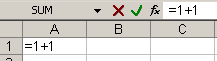3. Press Enter and notice how cell A1 changes from your formula to the result!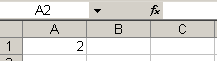This may seem simple, but there are a some very important things you should get out of this example. When you start off a cell entry with the equal sign "=" you are telling Excel that you want it to evaluate the following formula.

In our case we had a simple "1+1" we wanted Excel to solve for us. You can do this for addition, subtraction, multiplication, division and any other operation you can think of.

Remember, if you do not start your entry with the equal sign, then Excel will not evaluate the cell!

Using Cells to Create Dynamic Formulas

The most powerful aspect of Excel is not the simple calculator abilities we describes in our first formula example, but rather the ability to take values from cells to be used in your formulas.

Let's set up a basic sales spreadsheet to help explain this topic.

1. In cells A1-D4 enter the following information: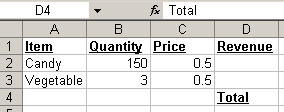Notice: that cell D2 and D3 are blank, but should contain the amount of money from selling 150 candy items and 3 vegetables. By referencing the Quantity and Price cells we will be able to do this! Let's begin with Candy.
2. Note:It is very important to follow these steps exactly without interruptions! Select cell D2, candy's "revenue", and type the equal sign "=" to begin your formula.
3. Left-click on cell B2, Candy's Quantity and notice your formula is now "=B2"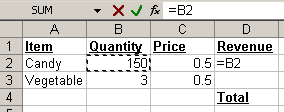4. We want to multiply Quanity(B2) by Price(B3) so enter an asterisk (*)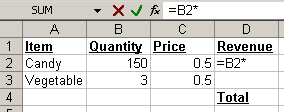5. Now left-click on Candy's Price (C2)to complete your formula!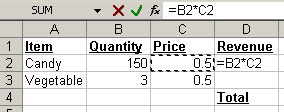6. If your formula looks like ours then press Enter, otherwise you can manually enter the formula "=B2*C2". However, we really think it is easier and preferred to click on cells to reference them, instead of entering that information manually.
7. After you pressed Enter your Candy Revenue cell should be functioning properly and contain the value 75.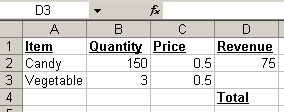8. Using your newly gained knowledge please complete Vegetable's Revenue by repeating steps 2-7 for Vegetable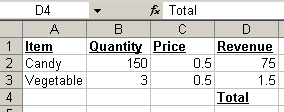10. Cheatsheet: If you are having trouble creating the formula for Vegetable's Revenue it is "=B3*C3"

Advanced Excel Formulas: Using Formulas in Formulas

Now that we have created separate revenues for both Candy and Vegetable it would be nice to somehow combine these two values to get the Total Revenue. Although both Vegetable Revenue and Candy Revenue contain formulas, we can still use these cells as we have been doing and add them together to get our total.

1. Select cell D5 (directly below "Total")
2. Type the equal sign "="
3. Left-click cell D2
4. Type the plus sign "+"
5. Left-click cell D3. Cell D5 should now contain this formula "=D2+D3":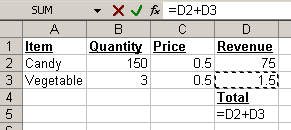6. Press Enter to complete your Total Revenue!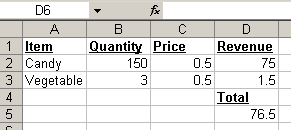Found Something Wrong in this Lesson?

Report a Bug or Comment on This Lesson - Your input is what keeps Tizag improving with time!

 Web Reference HTML Reference CSS Reference CSS Examples PHP Examples Help Tizag Grow Link to Tizag Tizag.com Store
 Advertise Here More Tutorials! Microsoft Office Tutorials Artist Tutorials